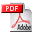The mixer stage needs some explanations...

Here is the schematics of this stage
• L1 and L2 are the tubes used in this stage, the tuning is made by the varcap CV1, in parallel with loop antenna, but by varcap CV2 which tunes the local oscillator frequency, too.
• T2 is the oscillator transformer.
• T3 and T4 are IF transformers.
• T1 is the coupling transformer.
• C2 is the compensation condensator (neutrodyn)
• C3 and C4 tune T3 and T4 at IF frequency (42 kHz).

Let's see how this stage works:How it works...

The received signal is selected by the tuning circuit which is constitued by S1 and CV1.
The frequencies of this signal are greater than 500kHz (broadcasting band).
For theese frequencies, C1 is a short-circuit, the signal is then applied at grid of L1.

This grid is biased across the T3 coil, but for received frequencies, this coil has a very high impedance, so the signal can't sink to the -C battery.

The signal is amplified by L1 and is present on its anode; the anodic circuit for the current passing through C4, which has a very low impedance for received frequencies.

The signal is then entirely tranmistted by T1 at the modulation tube, L2.

2. local oscillation signalHow it works...

One important thing is that it's the second harmonic of oscillator signal which is used.

This manner allows oscillator signal and received signal are not close each other, so, risk of oscillation stop doesn't exist.

Then, the oscillator frequency is greater than 250 kHz, and its second harmonic is greater than 500 kHz, too.

So, the C3 impedance is low and the tube L2 is powered by +B battery.
The T2 transformer makes the reaction of L2.

Note that the LO signal can easily pass through T1 secundary coil.

3. IF signalHow it works...

The 42 kHz beat appears in anodic circuit of L2 because this tube oscilles at local frequency (and second harmonic is present too) and is modulated by received signal via transformer T1.

The beat obtained from fundamental of oscillator and received signal is out of range, the it's permitted not considerate it.

At this frequency of 42kHz, the impedances of the transformers T3 and T4 are very high, and:

• T2 secundary impedance is very low,
• C1 impedance is very high.

The 42kHz beat then appears on the extremities of the circuit tuned by C3, and then is transmitted at the gris of L1 via T3.
In other words, L1 has on its grid the antenna signal AND the IF signal.

The 42kHz component is amplified by L1 et appears on its anode.
The primary coil of T1 has a very low impedance at 42 kHz, the signal is then entirely present on circuit tuned by C4.

The amplified IF signal is the transmitted by T4 on the next IF stage, like classic manner.

The condensator C2 gets the amplified IF signal from L1 and puts it on the grid of L1, with an attenuation and a phase in accordance with tranformation ratio of tapped coil of T3.

So, the internal capacity Cag of tube L1 (between anode and grid) is compensated (neutrodyn)